# Why does this not work? Projectile Motion problem for Dynamics Class

DioMiner
Thread moved from the technical forums to the schoolwork forums
TL;DR Summary: I want to mainly figure out where in the problem solving I went wrong. I understand the correct answer (since I looked it up), but to me, it does not make any sense.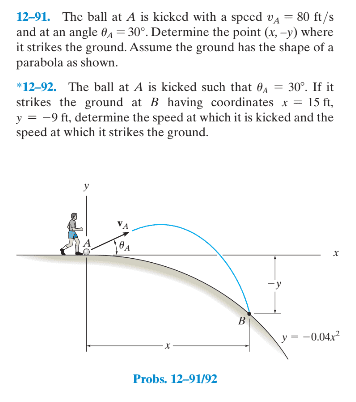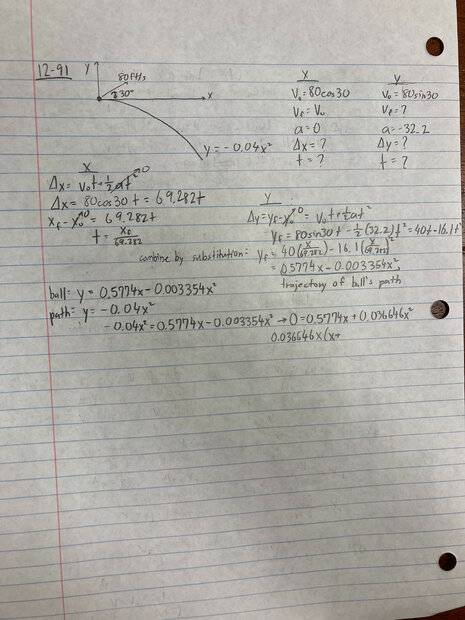I am honestly stumped at this point. Online solutions say that my equation y = 0.5774x-0.003354x^2 should instead be -y = 0.5774x-0.003354x^2 since "the change in y is negative"...
However, would the value y just be solved to be a negative value if all calculations are done correctly? I used a graphing calculator to check as well and the function, y = 0.5774x-0.003354x^2 is extremely different from how I imagine the projectile motion of this ball to look. Did I make a mistake somewhere while eliminating the parameter t from the equations? Or was there an assumption I made in solving that cannot be made? Thank you for the help in advance :).

Homework Helper
Gold Member
Your approach is correct, however your expression -0.04x2= 0.5774x -0.003354x2 troubles me because its non-zero solution is negative and that can't be. Probably you made a mistake somewhere in your calculation of all these numbers.

Try solving it using symbols all the way to the end. Then substitute the numbers at the very end. A symbolic representation of the answer would be easier to troubleshoot. Start by writing ##t=\dfrac{x}{v_0\cos(30^o)}## and replace that in ##y=v_0\sin(30^o) t-\frac{1}{2}gt^2## for starters.

Gold Member
That is curious. ##y## would go negative after ##\approx 172 \rm{ft} ##, but if you try to solve it as

$$-0.04 x^2 = \tan \theta x - \frac{1}{2}g\frac{x^2}{v^2 \cos^2 \theta}$$ which seems like the LHS must be a negative value you run into some issues. The method of factorization in the solution seems to be choosing the undesirable root that you would get plugging a negative value for ##y##

$$x \left( \tan \theta + \left( 0.04 - \frac{1}{2} \frac{g}{v^2 \cos^2 \theta} \right)x \right) = 0$$

When I solve that I get a solution for ##x## which is negative and far too small? Strange.

Last edited:
Gold Member
When ##y = 0 ## for the ball at ##x=172 ft##, the hill is 1,183 ft below the ball

$$y_h = -0.04 x^2 = -0.04 \cdot 172^2 = -1,183 \rm{ft}$$

Something is off. I'm pretty sure this means the ball never touches the hill using these figures (except at the origin).

Last edited:
•jack action
Homework Helper
Gold Member
Something is surely off. Is it possible with these numbers the ball never touches the hill?
Yes. Here is a graph of the two parabolas. It seems that the numbers are bad.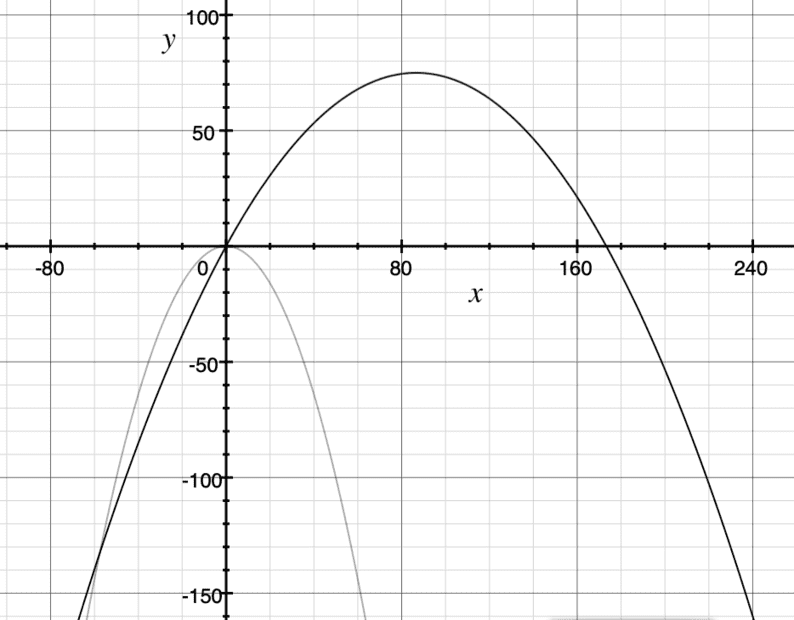•SammyS and erobz
Gold Member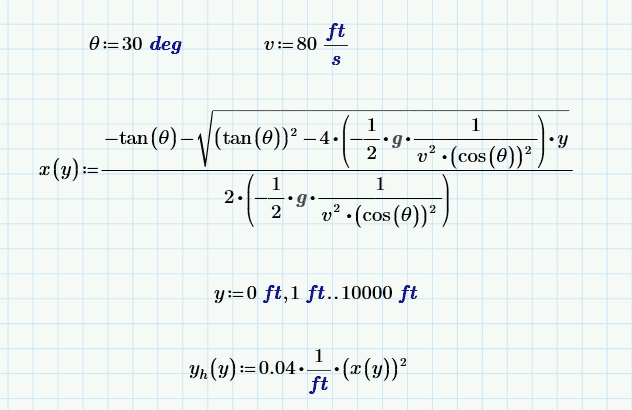There is the ##x## coordinate of the ball as a function of its height below the datum, ##x(y)##.

##y_h## is the hill elevation below the datum

Here is a plot of ## y(h) - y ## vs ##y##: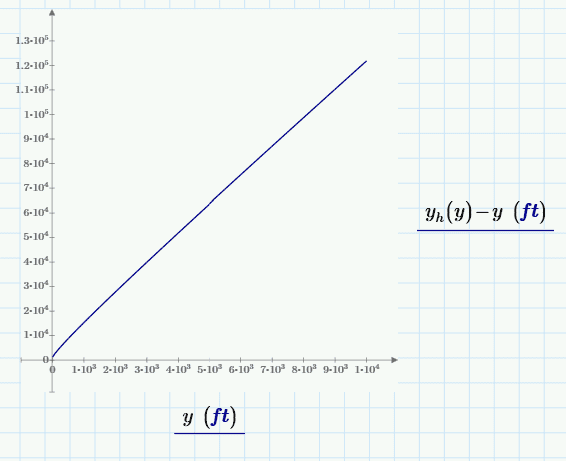The difference between them appears to be increasing without bound.

Gold Member
Yes. Here is a graph of the two parabolas. It seems that the numbers are bad.

View attachment 321316
Phew...thought I was loosing it!The OP said they had the solution. Maybe that is the actual problem though!! It probably says in the back of the book, "the ball only ever touches the hill at the origin".Homework Helper
Gold Member
Apparently, this is problem 12-91 from Engineering Dynamics from Hibbler 14e with initial speed 80 ft/s.
But from a solution I found online from a similar problem 12-106 from 13e has the initial speed of 8 ft/s.
I think it's a typo or else a poor attempt to change the input.

The following "solution" obtains a number... but, as many of you have found,
the projectile trajectory with the given numbers doesn't match
the diagram shown in the image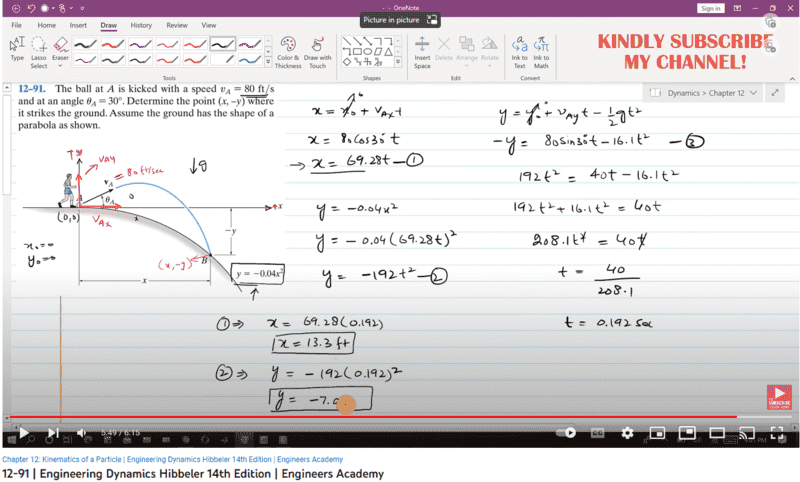vs.

issuu.com/manaren/docs/engineering_mechanics_dynamics_13th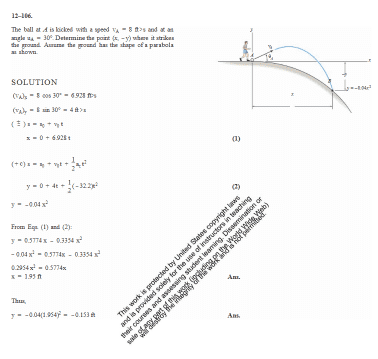To see what is going on, I made a desmos file
to solve the problem with various combinations.
https://www.desmos.com/calculator/t14gpeohny

The parabolic hill is in black.

The physical trajectory is the "CAP"-parabola in red.

This is for 8 ft/s (12-106 in 13e)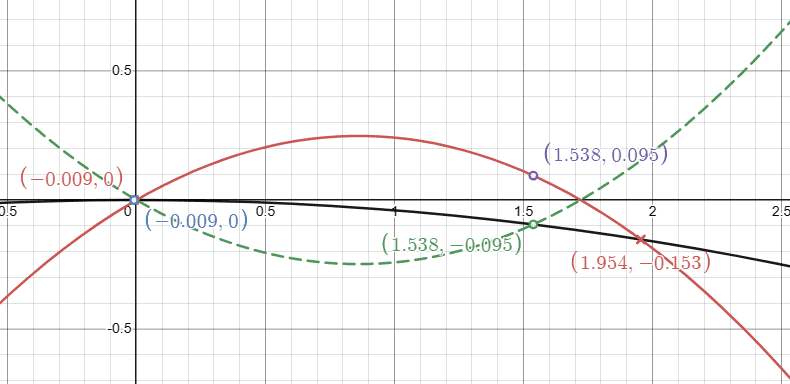This is for 80 ft/s (12-91 in 14e).
The "solution" uses the unphysical green-dashed CUP-parabola (with the (-y) nonsense).
Note that one must zoom out.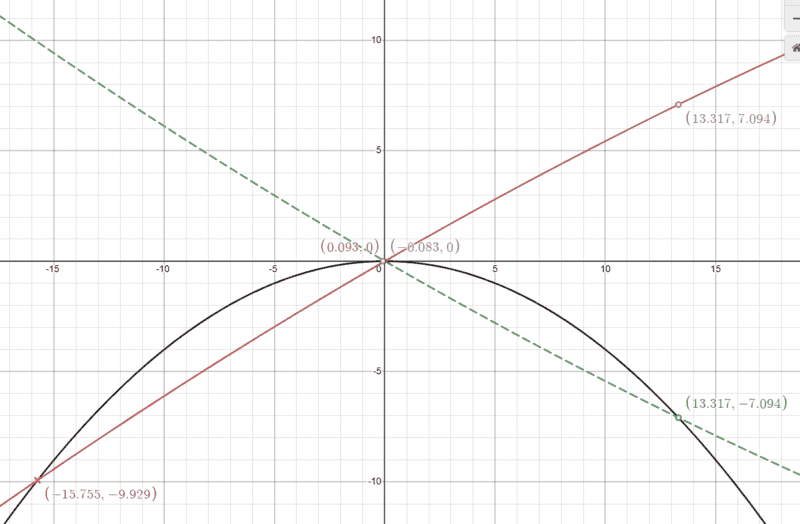DETAILS:

I used desmos's regression to solve a system of equations.
• For 8 ft/s (12-106 in 13e), the correct solution is ##X_{CAPpos}=1.95 ft##.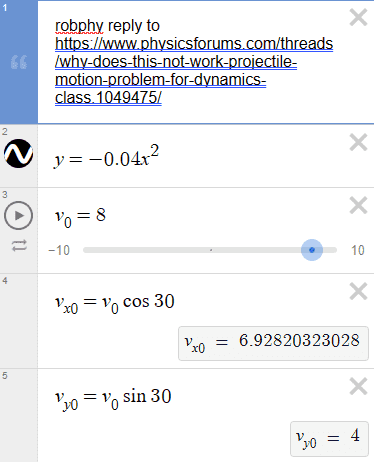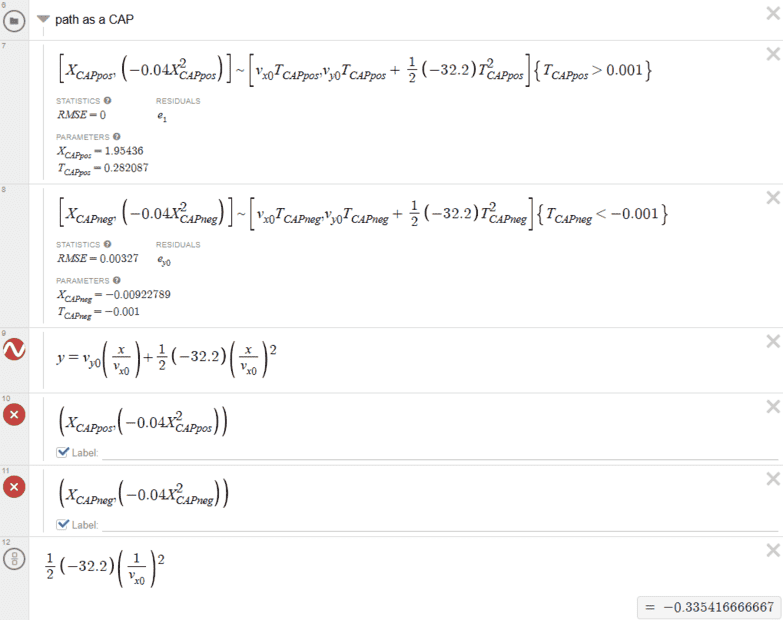• For 80 ft/s (12-91 in 14e) , (there is no physical solution),
but the incorrect "solution" gets their value for ##t>0## as ##X_{CUPpos}=13.31 ft##.
by using the unphysical CUP-parabola in green.... from the (-y) nonsense .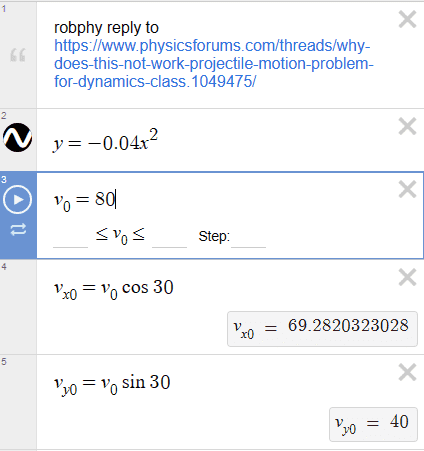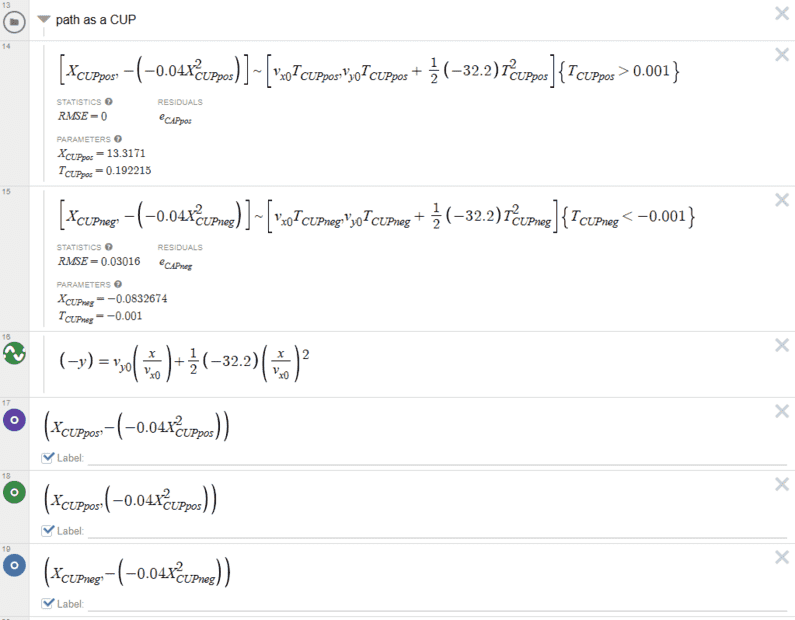So, as I said,
I think it's a typo or else a poor attempt to change the input.

Last edited:
••pinball1970, DioMiner, erobz and 1 other person
Homework Helper
Gold Member
The ball's trajectory equation is $$y=\tan\!\theta~x-\frac{1}{2}\frac{gx^2}{v_0^2\cos^2\!\theta}.$$The parabolic equation for the ground is ##y=-a^2x^2## (here ##a=0.2~\rm{m}^{-1/2}##). Setting the two equation equal, we find two solutions for horizontal position ##x## where the two parabolas intersect
1. ##x=0##, the initial position which we knew all along.
2. ##x=\dfrac{\tan\!\theta}{\frac{g}{2v_0^2\cos^2\!\theta} -a^2}.##
The value of ##x## must be positive which sets the following criterion for a solution to exist
$$\frac{g}{2v_0^2\cos^2\!\theta} >a^2 \implies av_0\cos\!\theta <\sqrt{\frac{g}{2}}.$$Here (ignoring units)

##av_0\cos\!\theta=0.2\times 80\times \frac{\sqrt{3}}{2}=13.9##
##\sqrt{\frac{g}{2}}=\sqrt{\frac{32}{2}}=4##

The criterion is not satisfied.

•hutchphd and erobz
Homework Helper
Gold Member
80 feet/second is much more reasonable speed for a typical soccer ball shot.
That equals 25 m/s or 55 mph approximately.

https://www.researchgate.net/figure...ore-impact-for-female-and-male_fig2_305080605
Then the problem needs to find a flatter (less pointy) parabola for the ground.

Compare the ##-0.04## in the ground-function ##y=(-0.04 ft^{-1} )x^2##
with the quadratic term in the trajectory ##y=\frac{1}{2}\frac{(-32.2 ft/s^2)}{ (80 ft/s\cos 30^\circ)^2 }x^2 =(-0.003354 ft^{-1})x^2##
Change the launch speed to 80, zoom out,
then try changing the -0.04 to something smaller
(say, -0.004, -0.0004, then probe near -0.003354 )
in my https://www.desmos.com/calculator/t14gpeohny .
To get the numerical solutions, you have to modify all the equations with your new value (I should make it a slider).

Here is an updated version:
https://www.desmos.com/calculator/hwgcbo8n8y

Last edited:
•Lnewqban and SammyS
DioMiner
My my, (sorry for the slow response, I've been a bit busy)
I am quite astonished with the amount of responses and I thank you all for helping me with this problem. I had been scratching my head over it for hours throughout the day when I tried. Thank you so so much for the attempt and everything has been extremely informative.

Initially my thoughts were exactly that of the latter end of this conversation, thinking that the ball simply doesn't hit the hill and that's the end of the problem... Thank you for the confirmation. I am glad that I'm not going crazy with this whole thing.
(I was just extremely confused because all online solutions set y as a negative value and reach a solution, hence I talked about knowing what the solution is...)

I am definitely going to meet with my professor during office hours for this problem to see how he thinks about it, and see it from that perspective as well.

Once again, thank you so much for the help!

Last edited:
•Lnewqban
Homework Helper
Gold Member
2022 Award
solves the problem by setting y to a negative value but that doesn't quite make sense to me.
The confusion over the definition of y starts in the statement of the problem.
The diagram shows the distance below the starting point is "-y". Since a distance is positive, y must be a negative value. I.e. the positive direction for the y coordinate is up.
This is confirmed by the parabola being given as y=-0.04x2.
The landing point is denoted (x,-y). If the positive direction for y is up, the y coordinate of the landing point must be negative, but since that y value itself is negative, (x,-y) is above the x axis!

The simplest fix is to specify the landing point as (x, y). The trajectory equation then becomes ##y=v\sin(\pi/6)t-gt^2/2##, instead of -y.

DioMiner
The landing point is denoted (x,-y). If the positive direction for y is up, the y coordinate of the landing point must be negative, but since that y value itself is negative, (x,-y) is above the x axis!
I understand that the landing point is denoted as (x,-y), and how the positive direction points up.
However, I still can't quite grasp why my approach concludes that there is no solution to the problem. Does the function y=-0.04x^2 not actually represent the hill? Or does the equation calculated from the parametric functions not actually represent the trajectory of the ball? If this is the case, I don't understand why.
Lets say that for this problem, the landing point was denoted as (x,y)... then would the answer instead be no solution?

PS: My professor responded to my email only saying to replace -y into my y equation... that it should be -y=-0.04x^2.

Homework Helper
Gold Member
2022 Award
However, I still can't quite grasp why my approach concludes that there is no solution to the problem.
Because there is no solution. As @robphy showed in post #11, the two parabolas do not intersect for t>0.
My professor responded to my email only saying to replace -y into my y equation... that it should be -y=-0.04x^2.
Obtaining what happens to look like a valid solution by deliberately using the wrong equation doesn't sound smart.

You avoided all this -y confusion by saying:
• the hill parabola is ##y=-0.04x^2##
• the landing point is ##(x,y)##
• the equations of motion are ##y=v\sin(\theta)t-\frac 12gt^2##, ##x=v\cos(\theta)t##
which is unarguably correct.

When you say "professor", do you just mean teacher, or has this twit actually been awarded a chair?

DioMiner
When you say "professor", do you just mean teacher, or has this twit actually been awarded a chair?
I am a university student and this class is ME 271: Engineering Mechanics - Dynamics (This homework is from early chapters talking about projectile motion so its not too convoluted). The response was indeed from my professor for this course... Maybe he didn't quite think it through? It was a very brief response that didn't have any justification to be fair.

Homework Helper
Gold Member
2022 Award
I am a university student and this class is ME 271: Engineering Mechanics - Dynamics (This homework is from early chapters talking about projectile motion so its not too convoluted). The response was indeed from my professor for this course... Maybe he didn't quite think it through? It was a very brief response that didn't have any justification to be fair.
Has he seen your solution? I guess he must have to respond that way.
Try copying @kuruman's analysis in post #10 (except, I would use a instead of a2 and write the final condition with the a in the denominator inside the square root, i.e. as g/(2a)). And accompany it with a plot illustrating the divergent parabolas.

•Lnewqban
DioMiner
Has he seen your solution? I guess he must have to respond that way.
Try copying @kuruman's analysis in post #10 (except, I would use a instead of a2 and write the final condition with the a in the denominator inside the square root, i.e. as g/(2a)). And accompany it with a plot illustrating the divergent parabolas.
Ok, I will try that out. Thanks so much for the help! This problems been quite annoying because of how the solution is given out as.

•Lnewqban
Homework Helper
Gold Member
Ok, I will try that out. Thanks so much for the help! This problems been quite annoying because of how the solution is given out as.
Maybe your professor for this course will profit from solving the problems he assigns beforehand. If he gets stuck, he can always seek help from PF, but please don't suggest that to him if you know what's good for you.••nasu and erobz
Gold Member
Maybe your professor for this course will profit from solving the problems he assigns beforehand. If he gets stuck, he can always seek help from PF, but please don't suggest that to him if you know what's good for you.Rough crowd...